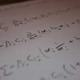• 150

# TrigonometryTrigonometry was first used by a Greek mathematician, Hipparchus, he mainly used trigonometry for astronomy. He then worked on defining chords sine and cosine. He used different ratios and recorded them on tablets. This was the beginning of Trigonometry.
• 160

# Rhind Papyrus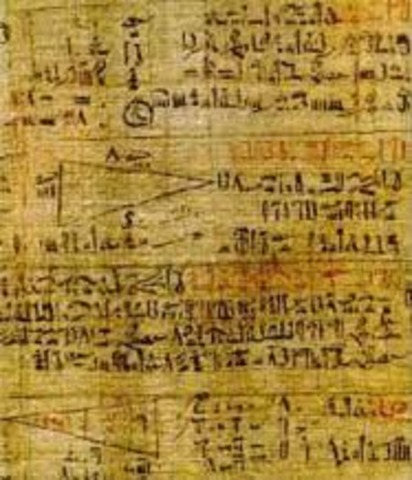This was one of the first math books that was created around 1600 BC. Created by the Egyptians this artifact has problems in geometry, arithmetic, fractions, and sequences. There are 84 problems that contain fraction and algerbra problems.
• 200

# Egyptian MathematicsHieroglyphic numerals were introduced in 2000BC. The system was a base ten system where one was represented by a stroke, ten a heel bone, a coil of rope for hundred, a lotus flower for one thousand, the pointing finger for ten thousand, a tadpole for one hundred thousand, and an astonished man for one million. The Pyramids were also constructed during this time demonstrating the knowledge of geometry as well.
• 287

# Archimedes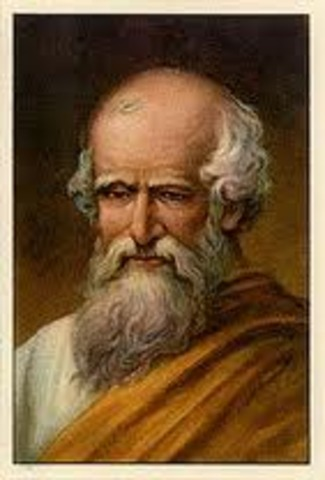Archimedes was born in 287 BC and was considered one of the greatest mathematicians in history. He contributed to the development in mathematics mostly in Geometry. Archimedes was also an accomplished engineer and an inventor. He also discovered how to find the volume of a sphere and helped find the exact value for Pi.
• 300

# The ElementsEuclid lived in Alexandria around 300 BCE and is most famous for his work, The Elements. It was contained text about geometry and number theory. This text was one of the most translated and circulated books that was ever written. It has been one of the most studied texts in the history of mathematics.
• 350

# Zero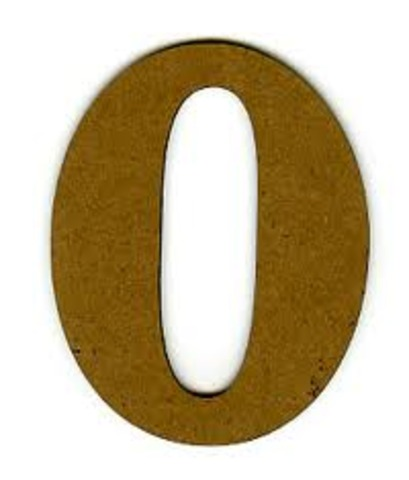The Babylonians were the first culture to invent the place value system. This is where the concept of zero started. The Mayans had a very complex number system and needed a placeholder. This lead to their invention of zero.
• 569

# PythagorasPythagoras was born in 569 BC and is best known for proving the Pythagorean Theorem. Pythagoras was a teacher, a philosopher, and a mystic. His thinking about mathematics and life was focused around numbers. The Sumerians used the Pythagorean Theorem earlier in their measurements, but Pythagoras proved that a2 + b2 = c2.
• Jan 1, 820

# The Quadratic FormulaIn his book, Hisab al-jabr w'al-muqabala, Al-Khwarizmi revealed basic theories of equations with examples and proofs including the earliest form of the quadratic equation which back then looked like this: x2 + 21 = 10x.
• Jan 1, 1072

# Cubic Equations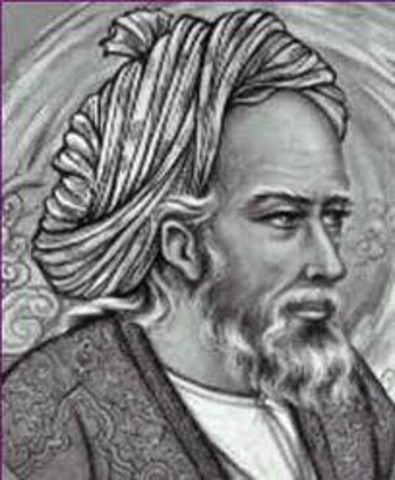Omar Khayyam was a Persian mathematician who gave a complete classification of cubic equations with positive roots and gives general geometric solutions to these equations found by means of intersecting conic sections. This lead him to solve the equation x3 + 200x = 20x2 + 2000.
• Jan 1, 1200

# Fibonacci SequenceThe Fibonacci sequence was invented by the Italian Leonardo Pisano Bigollo. This sequence was the outcome of a mathematical problem about rabbit breeding. He came up with this sequence: 1, 1, 2, 3, 5, 8, 13, 21, 34, 55, 89, 144... This is found by adding the two numbers that are next to each other to find the next number.
• Jan 1, 1545

# Negative NumbersThe concept of negative numbers has been around since the 200 BC and been a topic that has been argued about for thousands of years. It was Girolamo Cardano who for the first time described negative numbers as 'fictitious' and accepted the possibility of them being the solutions to equations. He described this in is book Ars Magna.
• Jan 1, 1572

# Imaginary NumbersImaginary numbers were first defined by Rafael Bombelli in 1572 as the square root of -1. Based off of his work, Descartes used the term “imaginary” number in 1637. However, the concept was invented much earlier by Gerolamo Cardano in the 16th century, but it was not widely accepted until the work of Leonhard Euler and Carl Friedrich Gauss
• # First ComputerScottish scientist John Napier created the first computer that worked with a system of sticks that would move in relation to each other and would perform multiplication automatically. This was called Napier's Bones.
• # Coordinate System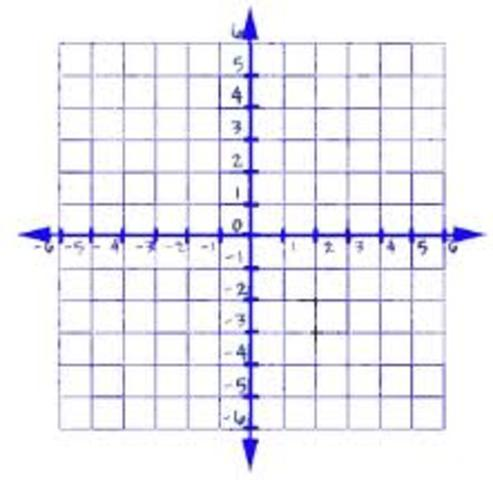René Descartes created the coordinate system that we use today in 1640. This system, also known as Cartesian Coordinate System, united geometry and algebra for the first time to create analytic geometry. The purpose is locating a point in space by giving its relative distance from perpendicular intersecting lines.
• # Probability Theory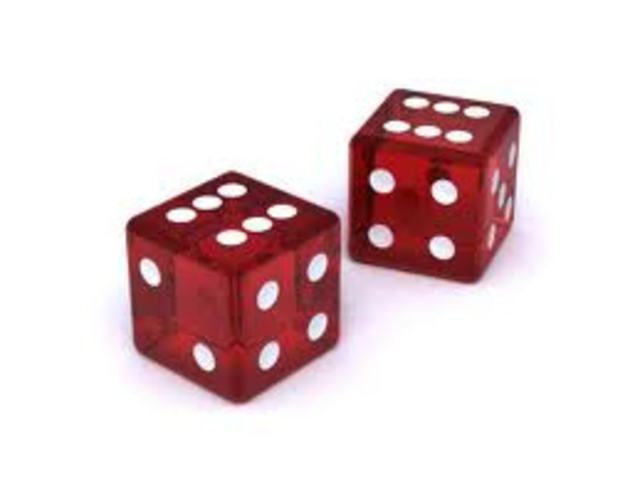Probability has developed over time to create the probability theory Chevalier de Mere proposed the “problem of points” in 1654.The “problem of points” involved trying to decide how to divide the stakes of an unfinished game in a fair way. Later Pascal and Fermat developed this dilemma more by comparing it to other gambling games.
• # Fermat’s Last TheoremFremat's Last Theorem states that x^n + y^n = z^n. In his notes, Fermat stated "I have discovered a marvelous proof of this, but the margin is too narrow to contain it." This theorem stumped mathematicians for almost 300 years. In 1993 Andrew Wiles announced he had found the proof to Fermat’s Last Theorem. In a few months he found a gap in his discovery and had to work on it until it was finally proven in 1994.
• # Pi Symbol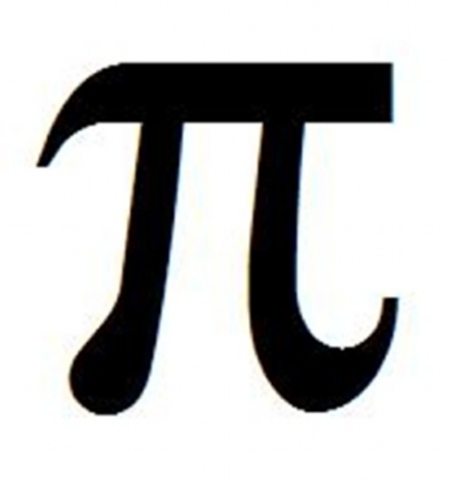Pi has been around since 2550 BC when the pyramids were built. The ratio of a pyramid was approximately two times Pi. Other cultures were close to finding the approximate value but none were as close as Archimedes who was the first to calculate the most accurate value of Pi. William Jones was the first to use the Greek symbol in 1707 and then it was adopted by Euler later and is now what we use today.
• # Metric SystemThe metric System was created in 1790 when The National Assembly of France requested that the French Academy of Sciences invent an accurate, standard system of weights and measures. The system they created was simple and scientific and was adopted by the French in 1795 AD.
• # InfinityGreeks used the idea of infinity to measure things that were too big to measure. It was Georg Cantor who actually discovered a described a way to do equations with infinite quantities. He described the idea of infinity by stating that a collection is infinite, if some of its parts are as big as the whole.
• # Albert EinsteinEinstein was known mostly for his work in Physics, but in 1905 he made the discovery that a large amount of energy could be released from a small amount of matter. This was expressed by the equation E=mc2. This contributed to the creation of the atomic bomb.